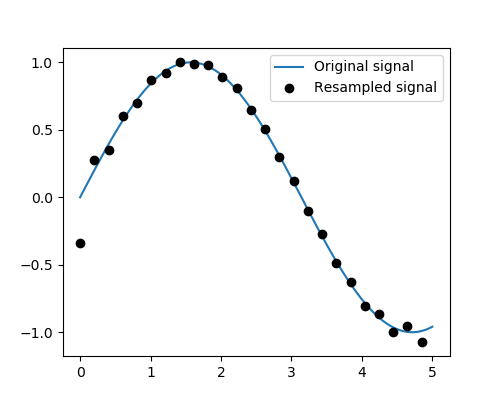# 1.6.12.3. Resample a signal with scipy.signal.resample¶

`scipy.signal.resample()` uses FFT to resample a 1D signal.

Generate a signal with 100 data point

```import numpy as np
t = np.linspace(0, 5, 100)
x = np.sin(t)
```

Downsample it by a factor of 4

```from scipy import signal
x_resampled = signal.resample(x, 25)
```

Plot

```from matplotlib import pyplot as plt
plt.figure(figsize=(5, 4))
plt.plot(t, x, label='Original signal')
plt.plot(t[::4], x_resampled, 'ko', label='Resampled signal')

plt.legend(loc='best')
plt.show()
```Total running time of the script: ( 0 minutes 0.018 seconds)

Gallery generated by Sphinx-Gallery